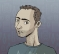## Problems extracting phase of dual FFTStarted by 4 years ago4 replieslatest reply 4 years ago147 views

I am performing dual FFT analysis on a system.

1) I generate a Log Sine Sweep signal and save it as my reference array

2) I then produce an inverse filter to correct for amplitude and save this as inverse array

3) I play this signal through my device under test, and record the result from a measurement microphone and save this as my measurement array.

4) I perform an FFT on all three arrays.

5) I multiply both the measurementFFT and the referenceFFT with the inverseFFT.

6) I then divide the corrected measurementFFT by the corrected referenceFFT

7) finally I perform an inverse FFT on this division product to get back to the time domain and this gives me my impulse response.

If I plot this I have a clear impulse response. If I try and extract the phase from this I have issues.

To do this I find the reference delay time by finding the max absolute value of the impulse. This will be the number of samples I need to delay the reference signal by if I am to extract minimal phase.

So, I go back to square one:

1) I zero pad the array

2) I then move the reference array so it is delayed by the impulse sample number. (The zero padding in the previous step results in zeros each side to equal the same length as the measurement array)

3) I then FFT both of these, repeating steps 4-6 from before

4) I then extract the phase from the impulse in the frequency domain, by using i = atan2(im(i), re(i)) for each value of the division product.

5) I then convert to degrees by multiplying each value by 180 and then dividing by pi.

If I plot this I end up with a lot of wraps in phase. Playing around a bit, I have discovered if I subtract my impulse delay time from the total count and use that as my new delay time, it gives the expected result. Have I missed something here. (ie if I have 32768 input samples, and my impulse is at sample 12, I would delay my reference by 32756 samples using the above method to give me the resultant phase I would expect).

Have I missed anything obvious?

[ - ]Hey there Samp17, I am not sure I do understand what the problem might be but I would suggest a couple of "tests" to acquire a better understanding. By delaying the reference signal by this (big amount of) time, looks like you could advance in time the measured signal to align them. Would you care to check this too?

One more thing you could try doing is to perform a delay in the frequency domain (adding a phase offset to all bins) and see if you get the same results. Again, I would suggest trying it both ways (delay/advance both signals and check the results).

Finally, just a side-note... You could possibly try to find the peak from the squared impulse response (energy method), which in close to ideal situations will yield similar (if not the same) results, but in some situations may slightly improve the noise robustness (beware, it is still quite susceptible to noise like the absolute impulse response method).

[ - ]just curious : are you trying to do speaker or room eq ?

[ - ]